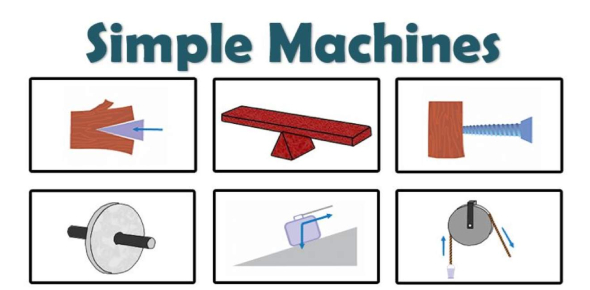# Simple Machines Quiz: Trivia Test

29 Questions | Attempts: 257
ShareSettings.

• 1.
What is any action that alters a body's state of rest; effort; pressure applied?
• A.

Friction

• B.

Force

• C.

• D.

Fulcrum

• 2.
What is the resistance between two forms of the matter when they move against one another?
• A.

Friction

• B.

Force

• C.

• D.

Fulcrum

• 3.
What produces friction?
• A.

Two materials moving apart

• B.

Two materials rubbing together

• C.

Two materials mixing together

• 4.
Does friction make work harder or easier?
• A.

Harder

• B.

Easier

• 5.
Does a rougher surface produce more or less friction?
• A.

More

• B.

Less

• 6.
Friction produces
• A.

Cold

• B.

Heat

• C.

Magnetism

• 7.
What is the support around which a lever turns called?:
• A.

Friction

• B.

Force

• C.

• D.

Fulcrum

• 8.
What is the bar that rests on the fulcrum called?
• A.

Plane

• B.

Lever

• C.

Wedge

• D.

Axle

• 9.
What does a lever do?
• A.

Makes it harder to move an object

• B.

Makes it easier to move an object

• 10.
What are a screw and staircase examples of?
• A.

Lever

• B.

Inclined plane

• C.

Wedge

• D.

Wheel and axle

• 11.
• A.

They are expensive

• B.

You can only use them on grass

• C.

You have to move the weight a greater distance

• 12.
What kind of lever has the fulcrum between the load and effort?
• A.

First class lever

• B.

Second class lever

• C.

Third class lever

• 13.
What kind of lever has the fulcrum at one end and the load between the fulcrum and effort?
• A.

First class lever

• B.

Second class lever

• C.

Third class lever

• 14.
A wheelbarrow and bottle opener are examples of?
• A.

First class lever

• B.

Second class lever

• C.

Third class lever

• 15.
A hammer, fishing rod and tweezers are examples of
• A.

First class lever

• B.

Second class lever

• C.

Third class lever

• 16.
When the fulcrum is at one end and the effort is between the load and fulcrum
• A.

First class lever

• B.

Second class lever

• C.

Third class lever

• 17.
A seesaw, balance scale, and pliers are examples of
• A.

First class lever

• B.

Second class lever

• C.

Third class lever

• 18.
What is another named for an inclined plane?
• A.

Wheel

• B.

Ramp

• C.

Wedge

• D.

Lever

• 19.
What lifts or lowers the objects along a slope using the least amount of force?
• A.

Lever

• B.

Inclined plane

• C.

Wedge

• D.

Wheel and axle

• 20.
What machine raises an object or splits it apart?
• A.

Lever

• B.

Inclined plane

• C.

Wedge

• D.

Wheel and axle

• 21.
Which machine uses less force to move the object, but takes a greater distance to move it?
• A.

Lever

• B.

Inclined plane

• C.

Wedge

• D.

Wheel and axle

• 22.
What machine is made of two wheels and moves heavy weights by reducing friction?
• A.

Lever

• B.

Inclined plane

• C.

Wedge

• D.

Wheel and axle

• 23.
What are wheels with teeth called?
• A.

Axle

• B.

Gear

• C.

Sharks

• D.

Spokes

• 24.
Where can you find gears?
• A.

Wagon, car, door knob

• B.

Bike, can opener, egg beater

• C.

Flag pole elevator

• 25.
What do elevators, flag poles, and window blinds use?
• A.

Gears

• B.

Pulley

• C.

Wheel and axle

## Related TopicsBack to top
×

Wait!
Here's an interesting quiz for you.